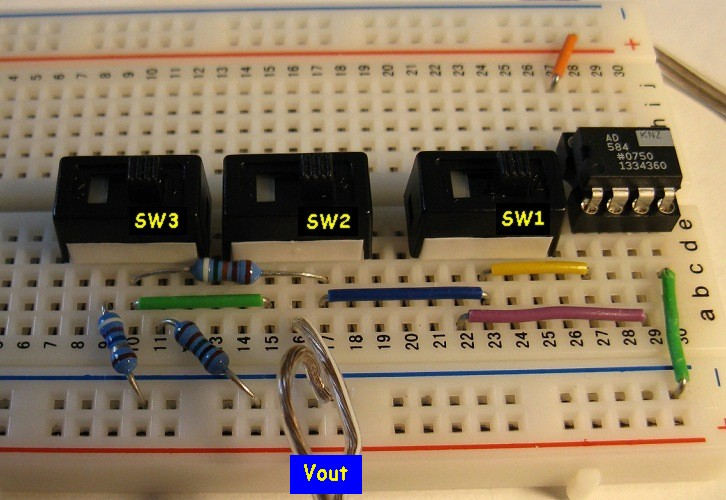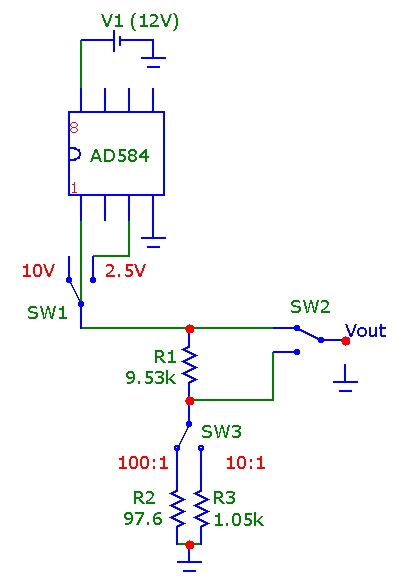## DC Voltage Calibration Source

March 08, 2009

Verifying the calibration of electronic measuring instrumentation such as voltmeters and oscilloscopes is important, particularly after many years of service. With available precision voltage reference ICs, it is easy to assemble a compact voltage reference. The AD584 precision programmable voltage reference is very easy to use and provides default voltage levels of 2.5, 5 and 10 VDC with an accuracy of ~ 0.1% for current loads of less than 10 mA which is adequate for verifying the calibration of high-impedance (Zin > 100 kohm) instruments.
For lower ranges, a simple voltage divider using resistors of 1% tolerance can easily extend the range down to the mV level, or higher precision resistors are available for greater accuracy in the lower ranges. The image below shows a simple breadboard implementation using the AD584KN voltage reference with 3 slider switches. Slider switch SW1 selects the 10.0 or 2.50 V range of the AD584. SW2 selects the direct voltage from the AD584 or a simple 2 resistor voltage divider network output. Slider switch SW3 selects either the 10:1 or 100:1 divider network. With this simple arrangement output DC voltages of 10V, 2.5V, 1V, 250 mV, 100 mV, 25 mV are available. The quiescent current draw of the AD584 is low enough ~ 1 mA that the circuit can be easily powered by a 12 VDC battery pack if required.In the circuit below, the available 1% resistors used were 9.53k, 1.05k and 97.6 ohms. The actual voltage divider values for these resistors are 9.92:1 and 101:1 respectively which are within the nominal 1% of the resistor tolerances. With the voltage divider switching in (SW2) and with the 10:1 selection (SW3) the load resistance of the text equipment should be greater than 100 kohm, which will ensure the voltage divider network ratio is accurate to better than 1%. For testing lower impedance equipment, the output of this circuit can be followed by a simple unity-gain opamp buffer circuit.